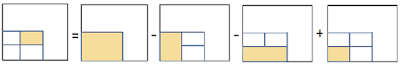## Integral Images / Summed Area Tables

In 1984, Crow proposed an image representation where each pixel value is replaced by the sum of all the pixels that belong to the rectangle defined by the lower left corner of the image and the pixel of our interest .
Even though more storage space may be required to save the image, the sum of every rectangle in the image/table can be calculated in constant time once the Integral Table is constructed. The summed area table (Integral Image) can be constructed in linear time O(n), where n is the number of pixels in the image, since one iteration through the entire image is enough to replace the pixel values inside the area table.Figure 1. This figure depicts the parameters of the following equation .

So, let’s assume the we have the above image and we would like to find the sum of the blue area defined by the pixels (x,y) and (x+Ly, y+Ly) included. Then the sum is given by:
sum = T(x+Lx, y+Ly) - T(x+Lx, y-1) - T(x-1,y+Ly) + T(x-1, y-1)Figure 2. Once the Integral Image is constructed, the sum of any rectangular area is calculated in constant time .

Where T(x,y) is the value in the Integral Table (T) with coordinates (x,y).

Example:
The following Figure shows an example of converting a table into into an Integral Table.Figure 3. This figure shows an example of converting a (a) Table  into an (b) Integral Table. The (c) shows the indexes used for guidance in the example later in the text for finding the sum of the shaded area.
Let's assume we have constructed the Integral Table (T) of Figure 3 and we want to find the sum of the shaded area. Then (x,y)=(1,1), Lx=2 and Ly=1. Therefore S is calculated as follow:

S = T(x+Lx, y+Ly) - T(x+Lx, y-1) - T(x-1,y+Ly) + T(x-1, y-1)

S = T(1+2,1+1)  – T(1+2,1-1) – T(1-1,1+1) + T(1-1,1-1)

S = T(3,2)– T(3,0)-T(0,2)+ T(0,0)

S = 78 – 10 – 15 + 1 = 54

and its correctness can be checked by summing the values of the shaded area in the non integral table in Figure 1a. As we can see below they are equal as the sum returned by the equation of the Integral table.
S = 10 + 11 + 12 + 6 + 7 + 8 = 54

## Integral Volumes

Integral Volumes is the extension of Integral images in 3D. Instead of a 2D image, we have a 3D Volume divided into cubes, named voxels. Each voxel value is replaced by the sum of all the pixels that belong to the cuboid defined by the lower left lower corner of the volume and the voxel of our interest. Then, at constant time we can calculate the sum of any cuboid area at constant O(n) time 
So let s assume that the value of the voxel (x,y,z). The sum (S) of the cuboid defined by (x,y,z) and
(x+lx,y+ly,z+lz) is given by:


where T(x,y,z) is the value of the voxel (x,y,z) inside the Integral Volume
S is the sum of the voxels inside the cuboid
define the length of the cuboid in the x, y z axes respectively.

The images are taken from the following article, which explains how Sum Area Tables, in other words Integral Images, are used in 3D (named Integral Volumes) to optimise reconstruction of polygon representations from voxelised data: https://www.mdpi.com/2072-4292/13/4/559/htm

Work Cited

 Crow, F.C. (1984, July). Summed-Area Tables for Texture Mapping. ACM, Computer Graphics, Volume 18, Number 3

 Miltiadou M, Campbell NDF, Cosker D, Grant MG. A Comparative Study about Data Structures Used for Efficient Management of Voxelised Full-Waveform Airborne LiDAR Data during 3D Polygonal Model Creation. Remote Sensing. 2021; 13(4):559. https://www.mdpi.com/2072-4292/13/4/559/htm